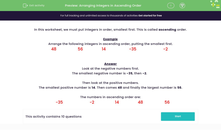# Arrange Integers in Ascending Order

In this worksheet, students will need to arrange positive and negative integers in ascending order of size.Key stage:  KS 2

Curriculum topic:   Number: Number and Place Value

Curriculum subtopic:   Use Negative Numbers

Difficulty level:#### Worksheet Overview

In this activity, we will be putting integers in order, smallest first. This is called ascending order.

Don't worry if you don't know the word integer - it simply means a whole number, either positive or negative.

Example

Arrange the following integers in ascending order, putting the smallest first.

 48 56 14 -35 -2

Look at the negative numbers first.

The smallest negative number is -35, then -2.

Then look at the positive numbers.

The smallest positive number is 14. Then comes 48 and finally the largest number is 56.

The numbers in ascending order are:

 -35 -2 14 48 56

If you find it hard to picture negative numbers, it can help to draw a number line with zero in the middle, positive numbers to the right of zero and negative numbers to the left of zero. The further to the right of zero you go, the larger the number. The further to the left of zero (into the negative numbers) you go, the smaller the number.

Let's have a go at some questions now.### What is EdPlace?

We're your National Curriculum aligned online education content provider helping each child succeed in English, maths and science from year 1 to GCSE. With an EdPlace account you’ll be able to track and measure progress, helping each child achieve their best. We build confidence and attainment by personalising each child’s learning at a level that suits them.

Get started